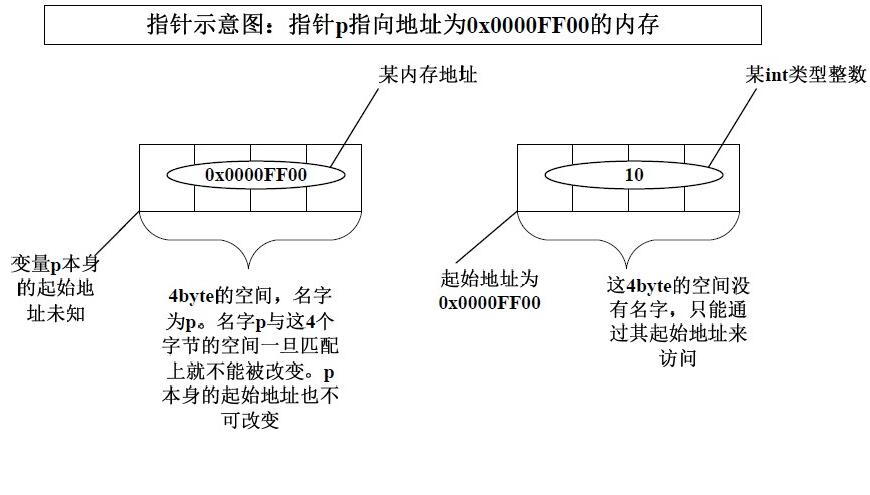2016
12-05

# C指针详解(经典,非常详细)

要了解指针,多多少少会出现一些比较复杂的类型,所以我先介绍一下如何完全理解一个复杂类型,要理解复杂类型其实很简单,一个类型里会出现很多运算符,他们也像普通的表达式一样,有优先级,其优先级和运算优先级一样,所以我总结了一下其原则:从变量名处起,根据运算符优先级结合,一步一步分析.下面让我们先从简单的类型开始慢慢分析吧:

```int p; //这是一个普通的整型变量
int *p; //首先从P 处开始,先与*结合,所以说明P 是一个指针,然后再与int 结合,说明指针所指向的内容的类型为int 型.所以P是一个返回整型数据的指针
int p; //首先从P 处开始,先与[]结合,说明P 是一个数组,然后与int 结合,说明数组里的元素是整型的,所以P 是一个由整型数据组成的数组
int *p; //首先从P 处开始,先与[]结合,因为其优先级比*高,所以P 是一个数组,然后再与*结合,说明数组里的元素是指针类型,然后再与int 结合,说明指针所指向的内容的类型是整型的,所以P 是一个由返回整型数据的指针所组成的数组
int (*p); //首先从P 处开始,先与*结合,说明P 是一个指针然后再与[]结合(与"()"这步可以忽略,只是为了改变优先级),说明指针所指向的内容是一个数组,然后再与int 结合,说明数组里的元素是整型的.所以P 是一个指向由整型数据组成的数组的指针
int **p; //首先从P 开始,先与*结合,说是P 是一个指针,然后再与*结合,说明指针所指向的元素是指针,然后再与int 结合,说明该指针所指向的元素是整型数据.由于二级指针以及更高级的指针极少用在复杂的类型中,所以后面更复杂的类型我们就不考虑多级指针了,最多只考虑一级指针.
int p(int); //从P 处起,先与()结合,说明P 是一个函数,然后进入()里分析,说明该函数有一个整型变量的参数,然后再与外面的int 结合,说明函数的返回值是一个整型数据
Int (*p)(int); //从P 处开始,先与指针结合,说明P 是一个指针,然后与()结合,说明指针指向的是一个函数,然后再与()里的int 结合,说明函数有一个int 型的参数,再与最外层的int 结合,说明函数的返回类型是整型,所以P 是一个指向有一个整型参数且返回类型为整型的函数的指针
int *(*p(int)); //可以先跳过,不看这个类型,过于复杂从P 开始,先与()结合,说明P 是一个函数,然后进入()里面,与int 结合,说明函数有一个整型变量参数,然后再与外面的*结合,说明函数返回的是一个指针,,然后到最外面一层,先与[]结合,说明返回的指针指向的是一个数组,然后再与*结合,说明数组里的元素是指针,然后再与int 结合,说明指针指向的内容是整型数据.所以P 是一个参数为一个整数据且返回一个指向由整型指针变量组成的数组的指针变量的函数.``````(1)int*ptr;
(2)char*ptr;
(3)int**ptr;
(4)int(*ptr);
(5)int*(*ptr);```

1.指针的类型

(1)int*ptr;//指针的类型是int*

(2)char*ptr;//指针的类型是char*

(3)int**ptr;//指针的类型是int**

(4)int(*ptr);//指针的类型是int(*)

(5)int*(*ptr);//指针的类型是int*(*)

2.指针所指向的类型

(1)int*ptr; //指针所指向的类型是int

(2)char*ptr; //指针所指向的的类型是char

(3)int**ptr; //指针所指向的的类型是int*

(4)int(*ptr); //指针所指向的的类型是int()

(5)int*(*ptr); //指针所指向的的类型是int*()

3.指针的值----或者叫指针所指向的内存区或地址

4 指针本身所占据的内存区

```char a;
int *ptr=(int *)a; //强制类型转换并不会改变a 的类型
ptr++;```

```int array={0};
int *ptr=array;
for(i=0;i<20;i++)
{
(*ptr)++;
ptr++；
}```

```char a="You_are_a_girl";
int *ptr=(int *)a;
ptr+=5;```

```#include<stdio.h>
int main()
{
char a=" You_are_a_girl";
char *p=a;
char **ptr=&p;
//printf("p=%d\n",p);
//printf("ptr=%d\n",ptr);
//printf("*ptr=%d\n",*ptr);
printf("**ptr=%c\n",**ptr);
ptr++;
//printf("ptr=%d\n",ptr);
//printf("*ptr=%d\n",*ptr);
printf("**ptr=%c\n",**ptr);
}```

&a 的运算结果是一个指针，指针的类型是a 的类型加个*，指针所指向的类型是a 的类型，指针所指向的地址嘛，那就是a 的地址。

*p 的运算结果就五花八门了。总之*p 的结果是p 所指向的东西，这个东西有这些特点：它的类型是p 指向的类型，它所占用的地址是p所指向的地址。

```int a=12; int b; int *p; int **ptr;
p=&a; //&a 的结果是一个指针，类型是int*，指向的类型是
//int，指向的地址是a 的地址。
*p=24; //*p 的结果，在这里它的类型是int，它所占用的地址是
//p 所指向的地址，显然，*p 就是变量a。
ptr=&p; //&p 的结果是个指针，该指针的类型是p 的类型加个*，
//在这里是int **。该指针所指向的类型是p 的类型，这
//里是int*。该指针所指向的地址就是指针p 自己的地址。
*ptr=&b; //*ptr 是个指针，&b 的结果也是个指针，且这两个指针
//的类型和所指向的类型是一样的，所以用&b 来给*ptr 赋
//值就是毫无问题的了。
**ptr=34; //*ptr 的结果是ptr 所指向的东西，在这里是一个指针，
//对这个指针再做一次*运算，结果是一个int 类型的变量。```

```int a,b;
int array;
int *pa;
pa=&a; //&a 是一个指针表达式。
Int **ptr=&pa; //&pa 也是一个指针表达式。
*ptr=&b; //*ptr 和&b 都是指针表达式。
pa=array;
pa++; //这也是指针表达式。```

```char *arr;
char **parr=arr; //如果把arr 看作指针的话，arr 也是指针表达式
char *str;
str=*parr; //*parr 是指针表达式
str=*(parr+1); //*(parr+1)是指针表达式
str=*(parr+2); //*(parr+2)是指针表达式```

```int array={0,1,2,3,4,5,6,7,8,9},value;
value=array; //也可写成：value=*array;
value=array; //也可写成：value=*(array+3);
value=array; //也可写成：value=*(array+4);```

```char *str={
"Hello,thisisasample!",
"Hi,goodmorning.",
"Helloworld"
};
char s；
strcpy(s,str); //也可写成strcpy(s,*str);
strcpy(s,str); //也可写成strcpy(s,*(str+1));
strcpy(s,str); //也可写成strcpy(s,*(str+2));```

*str 也是一个指针，它的类型是char *，它所指向的类型是char，它指向的地址是字符串"Hello,thisisasample!"的第一个字符的地址，即'H'的地址。注意:字符串相当于是一个数组,在内存中以数组的形式储存,只不过字符串是一个数组常量,内容不可改变,且只能是右值.如果看成指针的话,他即是常量指针,也是指针常量.

str+1 也是一个指针，它指向数组的第1 号单元，它的类型是char**，它指向的类型是char*。

*(str+1)也是一个指针，它的类型是char*，它所指向的类型是char，它指向"Hi,goodmorning."的第一个字符'H'

```int array;
int (*ptr);
ptr=&array;：```

int(*ptr);

sizeof(int(*))==4

sizeof(int)==40

sizeof(ptr)==4

```struct MyStruct
{
int a;
int b;
int c;
};
struct MyStruct ss={20,30,40};
//声明了结构对象ss，并把ss 的成员初始化为20，30 和40。
struct MyStruct *ptr=&ss;
//声明了一个指向结构对象ss 的指针。它的类型是
//MyStruct *,它指向的类型是MyStruct。
int *pstr=(int*)&ss;
//声明了一个指向结构对象ss 的指针。但是pstr 和
//它被指向的类型ptr 是不同的。```

ptr->a; //指向运算符，或者可以这们(*ptr).a,建议使用前者

ptr->b;

ptr->c;

*pstr； //访问了ss 的成员a。

*(pstr+1); //访问了ss 的成员b。

*(pstr+2) //访问了ss 的成员c。

```int array={35,56,37};
int *pa=array;
//通过指针pa 访问数组array 的三个单元的方法是：
*pa; //访问了第0 号单元
*(pa+1); //访问了第1 号单元
*(pa+2); //访问了第2 号单元```

int fun1(char *,int);

int (*pfun1)(char *,int);

pfun1=fun1;

int a=(*pfun1)("abcdefg",7); //通过函数指针调用函数。

```int fun(char *);
inta;
char str[]="abcdefghijklmn";
a=fun(str);
int fun(char *s)
{
int num=0;
for(int i=0;;)
{
num+=*s;s++;
}
return num;
}```

```float f=12.3;
float *fptr=&f;
int *p;```

p=&f;

p=(int*)&f;

```void fun(char*);
int a=125,b;
fun((char*)&a);
void fun(char*s)
{
charc;
c=*(s+3);*(s+3)=*(s+0);*(s+0)=c;
c=*(s+2);*(s+2)=*(s+1);*(s+1)=c;
}```

```unsigned int a;
TYPE *ptr; //TYPE 是int，char 或结构类型等等类型。
a=20345686;
ptr=20345686; //我们的目的是要使指针ptr 指向地址20345686

ptr=a; //我们的目的是要使指针ptr 指向地址20345686
//编译一下吧。结果发现后面两条语句全是错的。那么我们的目的就不能达到了吗？不，还有办法：
unsigned int a;
TYPE *ptr; //TYPE 是int，char 或结构类型等等类型。
a=N //N 必须代表一个合法的地址；
ptr=(TYPE*)a； //呵呵，这就可以了。```

```int a=123,b;
int *ptr=&a;
char *str;
b=(int)ptr; //把指针ptr 的值当作一个整数取出来。
str=(char*)b; //把这个整数的值当作一个地址赋给指针str。```

```char s='a';
int *ptr;
ptr=(int *)&s;
*ptr=1298；```

```char a;
int *ptr=&a;
ptr++;
*ptr=115;```TTF的家园-www.ttfde.top 个人博客以便写写东西，欢迎喜欢互联网的朋友一起交流!